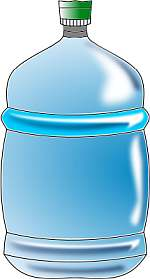# Quarts to Gallons

Instructions: Use this calculator to convert quarts to gallons, showing all the steps of the process. Please type the number of quarts you would like to convert in the form below:Type the number of quarts:

## Converting Quarts to Gallons

Quarts is a rather old unit of volume used, which still has some use, but largely due to a carryover of its past use.

In terms or order of magnitude, a quart is very similar in size to a liter, and metric units have gained popularity in recent decades, even in countries where the imperial system of units is the dominant one.

Converting into equivalent volume units is not a complicated task, but since there are some many different units (specially in the imperial system), it is kind of hard to memorize them all.## What is 1 gallon in quarts?

A gallon corresponds to exactly 4 quarts. Typically, the conversions within the imperial system have simple constants. It is when we convert between metric and imperial that we find more cumbersome conversion constants.

## How do you convert quarts to gallons

The conversion formula is really simple, but I would like to give the steps and rationale to derive it, so it is more likely that you will retain it in your mind.

## What are the steps for the conversion of quarts into gallons

1. First, you will use the observation that one gallon is equivalent to 4 quarts. This will the starting point of the proportion
2. Then, suppose you have Q quarts. You want to compute the number of gallons G that correspond to the given Q quarts
3. Now, we can establish a proportion: $$\displaystyle \frac{1}{4} = \frac{Q}{G}$$
4. Solving for G in this proportion, we find that the conversion formula from quarts to gallons is: $$G = \displaystyle \frac{Q}{4}$$

Observe that both quarts and gallons refer to U.S. quarts and gallons (as opposed to UK quarts and gallons).

## Why would you need to convert quarts to gallons

Necessity arises in different contexts. In the U.S. and other countries where the imperial units are dominant, there are a lot of "legacy" units that are not really too frequently used, but they are kept as a part of the whole unit legacy.

Gallons and quarts are broadly used still, and it is a practical tool to have this conversion calculator that will do the calculation for you, because even though the calculation is trivial, you may simply not remember it.### Examples: Converting quarts to gallons

Convert 12 quarts to gallons

Solution: First, we have to identify the amount of quarts we need to convert. We have in this case that we have to convert 12 quarts into gallons, so Q = 12. The conversion equivalence formula is:

$G = \displaystyle \frac{Q}{4}$

Therefore, we have to get Q = 12 and plug that into the conversion formula:

$G = \displaystyle \frac{Q}{4}= \displaystyle \frac{12}{4} = 3 \text{ gallons}$

### Examples: Another quarts to gallongs conversion

Convert 2 gallons to quarts

Solution: The equivalence conversion formula we have is:

$G = \displaystyle \frac{Q}{4}$

But in this case we have that G is given instead. Notice that

$G = \displaystyle \frac{Q}{4} \Rightarrow 2 = \displaystyle \frac{Q}{4}$ $\Rightarrow 2 \times 4 = Q$ $\Rightarrow Q = 8 \text { qt}$

In other words, we use the same formula to conduct a qt to gallon conversion instead.

## Other practical volume conversion tools

We have calculators to compute cups to ounces, and ounces to cups, which are very commonly requested conversions.

Also, you may be interested in a ounces to liters conversion, as well as in a liters to ounces conversion. These conversions are important because they form a bridge between metric and imperial units

Another perhaps less popular conversion tool is the cups to quarts conversion among others.